### Understanding Elementary Shapes-Solutions Ex-5.4

CBSE Class –VI Mathematics
NCERT Solutions
Chapter 5 Understanding Elementary Shapes (Ex. 5.4)

Question 1. What is the measure of (i) a right angle? (ii) a straight angle?
Answer: (i) The measure of a right angle is 90o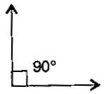(ii) The measure of a straight angle is 180o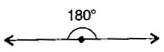Question 2. Say True or False:
(a) The measure of an acute angle < 90o
(b) The measure of an obtuse angle <90o
(c) The measure of a reflex angle > 180o
(d) The measure of one complete revolution= 360o
(e) If $m\mathrm{\angle }$A = 53o and $m\mathrm{\angle }$B = 35o then $m\mathrm{\angle }$A > $m\mathrm{\angle }$B.
Answer: (a) True, (b) False, (c) True, (d) True, (e) True
Question 3. Write down the measure of:
(a) some acute angles (b) some obtuse angles
(give at least two examples of each)
Answer: (a) Acute angles measure less than 90o e.g. 54,76o
(b) Obtuse angles measure greater than 90o. e.g. 105o, 154o
Question 4. Measure the angles given below, using the protractor and write down the measure: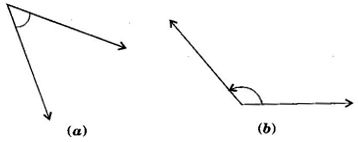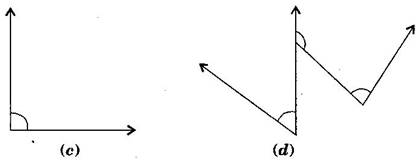Answer: (a) 40o   (b) 130o     (c) 90o   (d) 60o
other 2 angles i.e 90 degrees and 120 degrees
Question 5. Which angle has a large measure? First estimate and then measure:
Measure of angle A =
Measure of angle B =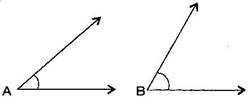Answer: $\mathrm{\angle }$B has a larger measure.
$\mathrm{\angle }$A = 40o and $\mathrm{\angle }$B = 65o
Question 6. From these two angles which have larger measure? Estimate and then confirm by measuring them: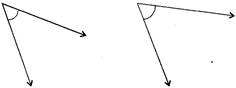Answer: The Second angle has a larger measure.
Question 7. Fill in the blanks with acute, obtuse, right or straight:
(a) An angle whose measure is less than that of a right angle is ________________.
(b) An angle whose measure is greater than that of a right angle is ________________.
(c) An angle whose measure is the sum of the measures of two right angles is _________.
(d) When the sum of the measures of two angles is that of a right angle, then each one of them is ________________.
(e) When the sum of the measures of two angles is that of a straight angle and if one of them is acute then the other should be ________________.
Answer: (a) acute angle, (b) obtuse angle, (c) straight angle, (d) acute angle, (e) obtuse angle
Question 8. Find the measure of the angle shown in each figure. (First estimate with your eyes and then find the actual measure with a protractor).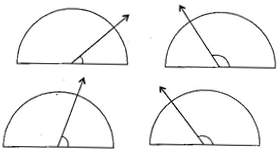Answer: (i) 30o (ii) 120o  (iii) 60o   (iv) 150o
Question 9. Find the angle measure between the hands of the clock in each figure: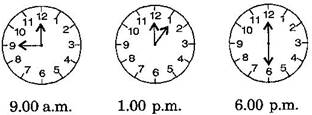Answer:  (i) 90o (Right angle) (ii) 30o (Acute angle) (iii) 180o (Straight angle)
Question 10. Investigate:
In the given figure, the angle measure ${30}^{\circ }.$ Look at the same figure through a magnifying glass. Does the angle becomes larger? Does the size of the angle change?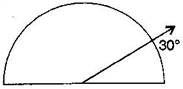Answer: No, the measure of angle will be same.
Question 11. Measure and classify each angle: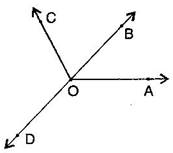Angle ∠$\mathrm{\angle }$AOB ∠$\mathrm{\angle }$AOC ∠$\mathrm{\angle }$BOC ∠$\mathrm{\angle }$DOC ∠$\mathrm{\angle }$DOA ∠$\mathrm{\angle }$DOB Measure Type
 Angle ∠$\mathrm{\angle }$AOB ∠$\mathrm{\angle }$AOC ∠$\mathrm{\angle }$BOC ∠$\mathrm{\angle }$DOC ∠$\mathrm{\angle }$DOA ∠$\mathrm{\angle }$DOB Measure 40o 130o 90o 90o 140o 180o Type Acute Obtuse Right Right Obtuse straight